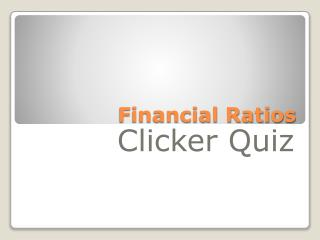# Financial Ratios - PowerPoint PPT PresentationDownload PresentationFinancial Ratios

Financial Ratios
Download Presentation## Financial Ratios

- - - - - - - - - - - - - - - - - - - - - - - - - - - E N D - - - - - - - - - - - - - - - - - - - - - - - - - - -
##### Presentation Transcript

1. Financial Ratios Clicker Quiz

2. Market Price Per Share • Earnings Per Share • A. Inventory Turnover • B. Accounts Receivable Turnover • C. Price Earnings Ratio • D. Debt to Equity What is this ratio?

3. Public’s confidence in the future growth of the company Price Earnings Ratio

4. Total Liabilities • Stockholder’s Equity • A. Inventory Turnover • B. Accounts Receivable Turnover • C. Price Earnings Ratio • D. Debt to Equity What is this ratio?

5. Proportions of debt to equity • Stockholders like it more than 1 since they will have more control • Creditors would like it less than 1 since the company is financed more by owners than creditors Debt to Equity Ratio

6. Cost of Goods Sold • Average inventory • A. Inventory Turnover • B. Accounts Receivable Turnover • C. Price Earnings Ratio • D. Debt to Equity What is this ratio?

7. How many times inventory has been bought and sold during the year. • 4.0 Times would mean it is sold once a quarter. 12 times is once a month Inventory Turnover

8. Sales on Account • Average Accounts Receivable • A. Inventory Turnover • B. Accounts Receivable Turnover • C. Price Earnings Ratio • D. Debt to Equity What is this ratio?

9. How many times a company converts its receivables into cash each year. • 52 times would be once a week – 7 days Accounts Receivable Turnover

10. Dividends Per Share • Market Price Per Share • A. Return on Assets • B. Dividend Payout • C. Return on Equity • D. Dividend Yield What ratio is this?

11. Return (dividends) on current market price of the stock Dividend Yield Ratio

12. Dividends Per Share • Earnings Per Share • A. Return on Assets • B. Dividend Payout • C. Return on Equity • D. Dividend Yield What is the ratio?

13. Portion of current earnings being paid out in dividends. Investors for dividends want this to be a large %. Investors seeking market price increase would like it to be small. Dividend Payout Ratio

14. Net Income • Average Total Assets • A. Return on Assets • B. Dividend Payout • C. Return on Equity • D. Dividend Yield What is the ratio?

15. How well the managers are using the assets to produce income • We are ignoring the difference in comparable companies loans to buy the assets Return on Assets

16. Net Income • Average Stockholder’s Equity • A. Return on Assets • B. Dividend Payout • C. Return on Equity • D. Dividend Yield What is this ratio?

17. Measures how well the company used the owner’s investments to earn income Return on Stockholder’s Equity

18. Current Assets – Current Liabilities • A. Current Ratio • B. Book Value • C. Quick Ratio • D. Working Capital What is the ratio?

19. Excess of current assets to current liabilities. Shouldn’t have too much because you are not making your money work for you or you might have borrowed too much and are paying too much interest. Working Capital

20. Cash, Marketable Securities, A/R, Short N/R • Current Liabilities • A. Current Ratio • B. Book Value • C. Quick Ratio • D. Working Capital What is the ratio?

21. Should be more than 1. Measures a company’s ability to meet obligations without having to liquidate inventory. Acid Test (Quick) Ratio

22. Current Assets • Current Liabilities • A. Current Ratio • B. Book Value • C. Quick Ratio • D. Working Capital What is the ratio?

23. Measures company’s short term debt paying ability. • 2 is a good number Current Ratio

24. Common Stockholder’s Equity • # of Common Shares Outstanding • A. Current Ratio • B. Book Value • C. Quick Ratio • D. Working Capital What is the ratio?

25. Amount to be distributed to holders of each share of common stock if all assets were sold at their balance sheet carrying amounts after all creditors were paid off. • Does not equal market value. • Market Value represents future whereas book value represents historical Book Value Per Share